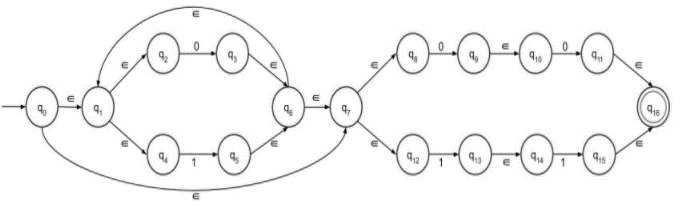# Construct ‚àà-NFA of Regular Language L = (0+1)*(00+ 11)

The ε transitions in Non-deterministic finite automata (NFA) are used to move from one state to another without having any symbol from input set Σ

ε-NFA is defined in five tuple

{Q, q0, Σ, δ, F}

Where,

• δ − Q × (Σ∪ε)→2Q

• Q − Finite set of states

• Σ − Finite set of the input symbol

• q0 − Initial state

• F − Final state

• δ − Transition function

## NFA without ε transition

NFA is defined in 5 tuple representation

{Q, q0, Σ, δ, F}

Where,

• δ − Q X Σ→ 2Q

• Q − Finite set of states

• Σ, − Finite set of the input symbol

• q0 − Initial state

• F − Final state

• δ − Transition function

NFA and NFA with epsilon both are almost the same; the only difference is their transition function.

Let’s consider the given language L = 0(0+1)*1

Rules for construction of ε-NFA are as follows −

Step 1 − NFA with epsilon for 0+ is given below −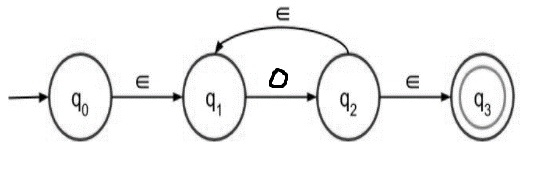Step 2 − NFA with epsilon for 0* is given below −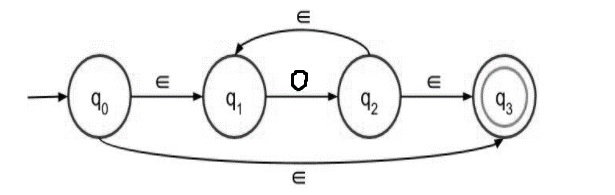Step 3 − NFA with epsilon for (0+1) is given below −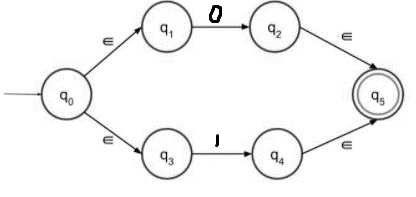The above transition diagram accepts either 0 or 1 as input. Those two paths lead to the final state.

Step 4 − NFA with epsilon for 01 is given below −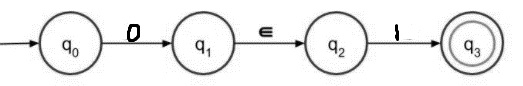For concatenation 0 must be followed by 1.

Step 5

ε-NFA for L = (0+1)*(00 + 11)

L = (0+1)*(00 + 11) is divided into two parts: (0+1)* and (00+11).

First construct the first part and then second part finally concatenate two parts to get the result.

First part − (0+1)*

With the help of step 3 we can easily construct (0+1)* as shown below −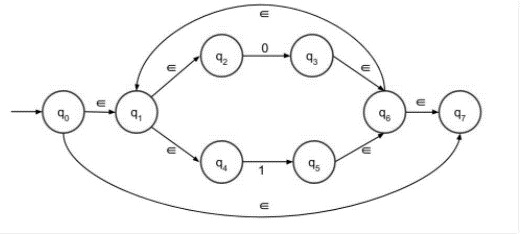Second part − (00+11)

The second part can be easily drawn with the help of step 4.

In step 4, consider 1 and 0 both are either 00 or 11. Both strings are connected by + sign.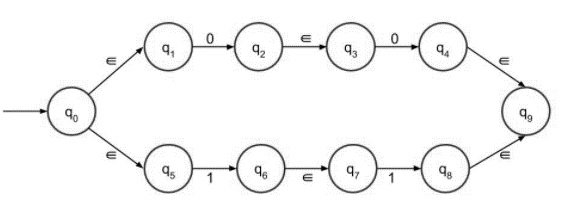The final NFA with epsilon move is as follows

Concatenate the first and second part,